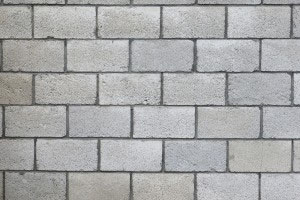SEARCH HOMEMath Central Quandaries & QueriesQuestion from Robert, a parent: I have a wall 40 ft. long and 4 ft. tall as well as another one that is 30 ft by 3.5 ft. tall that I am trying to build. how many 6 inch by 12 inch landscaping blocks do I need?Hi Robert,

your first wall is 40 ft by 4 ft so its surface area is $40 \times 4 = 160$ square feet. Your second wall has a surface area of $30 \times 3.5 = 105$ square feet. Thus you have a total surface area of $160 + 105 = 265$ square feet. Each block is half a foot by one foot so its area is $\large \frac12 \normalsize \times 1 = \large \frac12$ a square foot. Hence you will need at least $265 \times 2 = 530$ blocks. I say at least since there is bound to be some wastage. In particular if you arrange the blocks in the typical patternImage from http://gergsconstructionllc.com/concrete-blocks/

then every other row will require cutting a block. Can you do so and save the half block you cut off or do you loose half a block at each end?

PennyMath Central is supported by the University of Regina and the Imperial Oil Foundation.